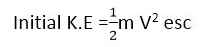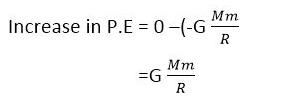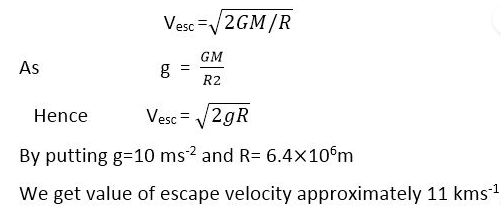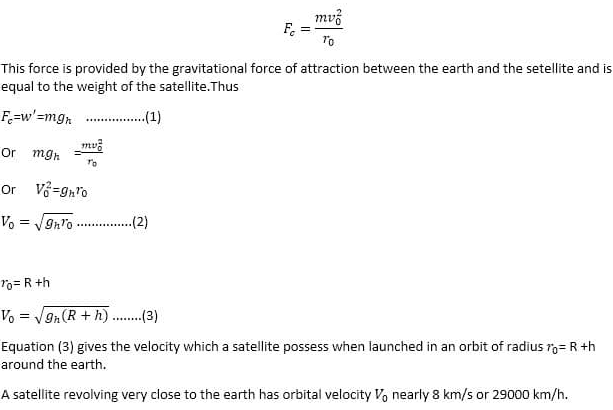Mechanics

# Relation between escape velocity and orbital velocity formula

The Basic Difference between Escape velocity and orbital velocity is: The velocity required by a body to escape from the earth’s gravitational field is called escape velocity. Its value is 11 km/s. Orbital velocity is the velocity required for the satellite to move in the earth’s orbit. Its value is 8 km/s or 29000 km/h.

## What is  Escape velocity?

“The velocity required by a body to escape from the earth’s gravitational field.”It is our daily life experience that an object projected upward comes back to the ground after rising to a certain height. This is due to the force of gravity acting downward. With increased initial velocity, the object rises to a greater height before coming back. If we go on increasing the initial velocity of the object,a stage comes when it will not return to the ground. It will escape out of the influence of gravity. The initial velocity of an object with which it goes out of the earth’s gravitational field is known as E.V.

## Escape velocity formula

The E.V. corresponds to the initial kinetic energy gained by the body, which carries it to an infinite distance from the surface of the earth.We know that the work done in lifting a body from the earth’s surface to an infinite distance is equal to the increase in its potential energy:Where M and R are the mass and radius of the earth respectively. The body will escape out of the gravitational field if the initial kinetic energy of the body is equal to the increase in potential energy of the body in lifting it up to infinity. Then## Orbital velocity

When the object in the earth’s orbit, its motion is known as orbital motion. The velocity by which the object moves in the earth’s orbit is called orbital velocity. It remains constant in the gravitational field.
The earth and some other planets revolve around the sun in closely circular paths. The artificial satellites launched by men also adopt a nearly circular course around the earth. This type of motion is called orbital motion. The formula for this velocity is given as:
Consider a satellite going around the earth in a circular path. The mass of the satellite is m and vº is the orbital speed. The mass of the Earth is M and rº represents the radius of the orbit. A centripetal force is required to hold the satellite in orbit which is given as:### One Comment

1. William Ferrigno says:

Great site. Registration needed ?